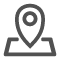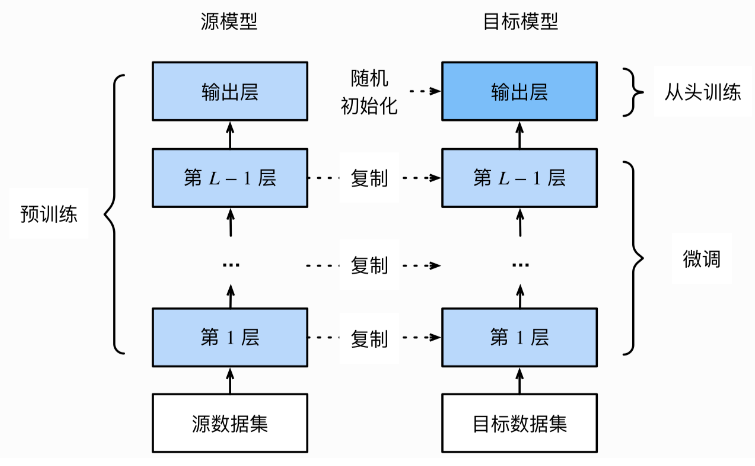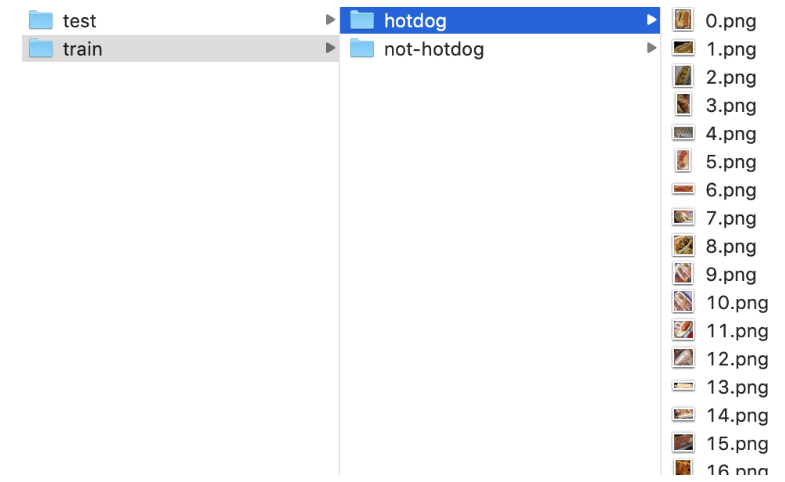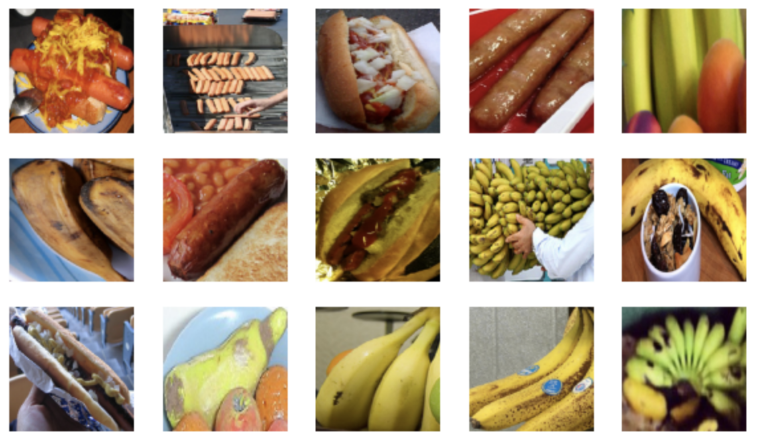# 什么是微调模型？微调模型实现热狗识别

## 微调的原理

1.在源数据集(如ImageNet数据集)上预训练一个神经网络模型，即源模型。

2.创建一个新的神经网络模型，即目标模型。它复制了源模型上除了输出层外的所有模型设计及其参数。我们假设这些模型参数包含了源数据集上学习到的知识，且这些知识同样适用于目标数据集。我们还假设源模型的输出层跟源数据集的标签紧密相关，因此在目标模型中不予采用。

3.为目标模型添加一个输出大小为目标数据集类别个数的输出层，并随机初始化该层的模型参数。

4.在目标数据集(如椅子数据集)上训练目标模型。我们将从头训练输出层，而其余层的参数都是基于源模型的参数微调得到的。## 热狗模型

```import tensorflow as tf
import numpy as np```

### 获取数据集```flow_from_directory(self, directory,
target_size=(256, 256), color_mode='rgb',
classes=None, class_mode='categorical',
batch_size=32, shuffle=True, seed=None,
save_to_dir=None）```

directory: 目标文件夹路径，对于每一个类对应一个子文件夹，该子文件夹中任何JPG、PNG、BNP、PPM的图片都可以读取。

target_size: 默认为(256, 256)，图像将被resize成该尺寸。

batch_size: batch数据的大小，默认32。

shuffle: 是否打乱数据，默认为True。

```# 获取数据集
import pathlib
train_dir = 'transferdata/train'
test_dir = 'transferdata/test'
# 获取训练集数据
train_dir = pathlib.Path(train_dir)
train_count = len(list(train_dir.glob('*/*.jpg')))
# 获取测试集数据
test_dir = pathlib.Path(test_dir)
test_count = len(list(test_dir.glob('*/*.jpg')))
# 创建imageDataGenerator进行图像处理
image_generator = tf.keras.preprocessing.image.ImageDataGenerator(rescale=1./255)
# 设置参数
BATCH_SIZE = 32
IMG_HEIGHT = 224
IMG_WIDTH = 224
# 获取训练数据
train_data_gen = image_generator.flow_from_directory(directory=str(train_dir),
batch_size=BATCH_SIZE,
target_size=(IMG_HEIGHT, IMG_WIDTH),
shuffle=True)
# 获取测试数据
test_data_gen = image_generator.flow_from_directory(directory=str(test_dir),
batch_size=BATCH_SIZE,
target_size=(IMG_HEIGHT, IMG_WIDTH),
shuffle=True)```

```import matplotlib.pyplot as plt
# 显示图像
def show_batch(image_batch, label_batch):
plt.figure(figsize=(10,10))
for n in range(15):
ax = plt.subplot(5,5,n+1)
plt.imshow(image_batch[n]）
plt.axis('off')
# 随机选择一个batch的图像
image_batch, label_batch = next(train_data_gen)
# 图像显示
show_batch(image_batch, label_batch)```### 模型构建与训练

Keras应用程序(keras.applications)是具有预先训练权值的固定架构，该类封装了很多重量级的网络架构，如下图所示：```tf.keras.applications.ResNet50(
include_top=True, weights='imagenet', input_tensor=None, input_shape=None,
pooling=None, classes=1000, **kwargs)```

include_top: 是否包括顶层的全连接层。

weights: None 代表随机初始化， 'imagenet' 代表加载在 ImageNet 上预训练的权值。

input_shape: 可选，输入尺寸元组，仅当 include_top=False 时有效，否则输入形状必须是 (224, 224, 3)(channels_last 格式)或 (3, 224, 224)(channels_first 格式)。它必须为 3 个输入通道，且宽高必须不小于 32，比如 (200, 200, 3) 是一个合法的输入尺寸。

```# 加载预训练模型
ResNet50 = tf.keras.applications.ResNet50(weights='imagenet', input_shape=(224,224,3))
# 设置所有层不可训练
for layer in ResNet50.layers:
layer.trainable = False
# 设置模型
net = tf.keras.models.Sequential()
# 预训练模型
# 展开
# 二分类的全连接层

```# 模型编译：指定优化器，损失函数和评价指标
loss='categorical_crossentropy',
metrics=['accuracy'])
# 模型训练：指定数据，每一个epoch中只运行10个迭代，指定验证数据集
history = net.fit(
train_data_gen,
steps_per_epoch=10,
epochs=3,
validation_data=test_data_gen,
validation_steps=10
)```
```Epoch 1/3
10/10 [==============================] - 28s 3s/step - loss: 0.6931 - accuracy: 0.5031 - val_loss: 0.6930 - val_accuracy: 0.5094
Epoch 2/3
10/10 [==============================] - 29s 3s/step - loss: 0.6932 - accuracy: 0.5094 - val_loss: 0.6935 - val_accuracy: 0.4812
Epoch 3/3
10/10 [==============================] - 31s 3s/step - loss: 0.6935 - accuracy: 0.4844 - val_loss: 0.6933 - val_accuracy: 0.4875```